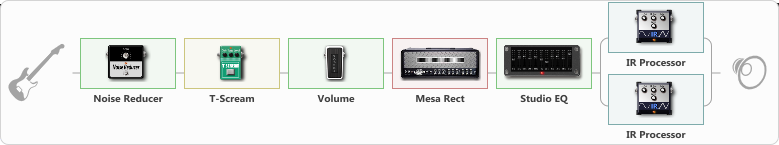# Messa Boogie

Discussion in 'ToneLib-GFX presets' started by levitation, May 4, 2020.

1. Messa Boogie

Preset name: Mesa Boogie

Pull me under Rytnem

Effects chain:Effect: "Noise Reducer" (Dynamics / Filter), active - "yes"
{
"Sens" = 57
"Mode" = Soft
}

Effect: "T-Scream" (Overdrive / Distortion), active - "yes"
{
"Drive" = 17
"Tone" = 97
"Level" = 41
}

Effect: "Volume" (Dynamics / Filter), active - "yes"
{
"Pedal Pos." = 18
"Pan" = 0
}

Effect: "Mesa Rect" (Amp simulators), active - "yes"
{
"Gain" = 73
"Bass" = 77
"Middle" = 61
"Treble" = 72
"Presence" = 33
"Master" = 76
"Level (dB)" = 7
}

Effect: "Studio EQ" (Dynamics / Filter), active - "yes"
{
"31 Hz" = 3
"62 Hz" = 3
"125 Hz" = 2
"250 Hz" = -2
"500 Hz" = -2
"1 kHz" = 0
"2 kHz" = 2
"4 kHz" = 3
"8 kHz" = 3
"16 kHz" = 1
"Level (dB)" = -3
}

Effect: "Splitter" (Dynamics / Filter), active - "yes"
{
"A-Bypass" = Off
"A-Pan" = -69
"A-Level" = 55
"B-Bypass" = Off
"B-Pan" = 70
"B-Level" = 55

'A' branch:
{

Effect: "IR Processor" (Cabinets), active - "yes"
{
"IR" = OH 412 MES-ST V60 160-M+
"Low Cut (Hz)" = 103
"Hi Cut (kHz)" = 20.0
"Mix" = 100
"Level (dB)" = 0
}
}
'B' branch:
{

Effect: "IR Processor" (Cabinets), active - "yes"
{
"IR" = OH 412 MES-ST V60+V70 JS-1
"Low Cut (Hz)" = 64
"Hi Cut (kHz)" = 6.0
"Mix" = 100
"Level (dB)" = 0
}
}
}

Note: You will need to download and install the ToneLib-GFX software to use the preset.

File size:
19.6 KB
Views:
2,907